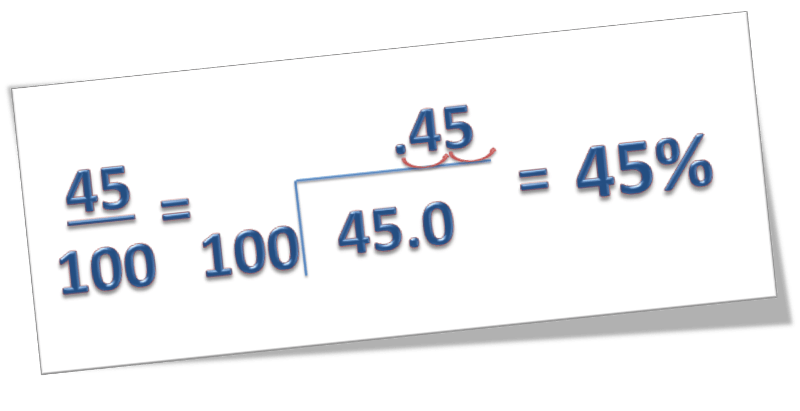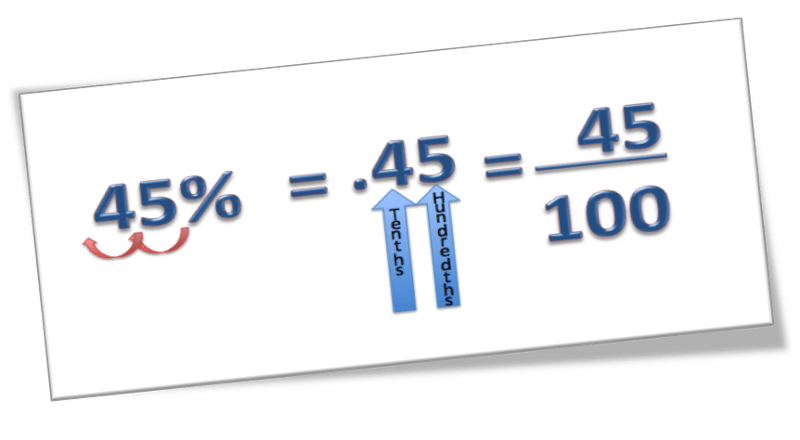# Fractions, Decimals and Percents

We can designate equivalent amounts of a whole by using a fraction, a decimal number, or a percent.Let’s practice.

1. Find the decimal equivalent of 51/100.
• To find the decimal equivalent of 51/100, we divide.   51/100 = 51 ÷ 100 =   0.51
2. Find the percent equivalent of 51/100.
• To change a decimal to a percent, we move the decimal point two places to the right.
• .51 = 51 percent or 51%

Use the table below to understand how to use fractions, decimals, and percents interchangeably:

#### Decimals to Percents

Move the decimal two places to the right and add a % sign.#### Decimals to Fractions

Use your place value information to write it over the appropriate denominator. (Tenths place will be over 10. Thousandths place would be over 1000.) Then reduce the fraction.#### Fractions to Decimals

Using a calculator or long division, divide the two numbers. You’ll be asked to round to a specific decimal place.#### Fractions to Percents

Convert to a decimal, then move the decimal two places to the right and add the % sign.#### Percents to Decimals

Move the decimal two places to the left and remove the % sign.#### Percents to Fractions

Change the percent to a decimal then use your place value information to write it over the appropriate denominator. (Tenths place will be over 10. Thousandths place would be over 1000.) Then reduce the fraction.(source)